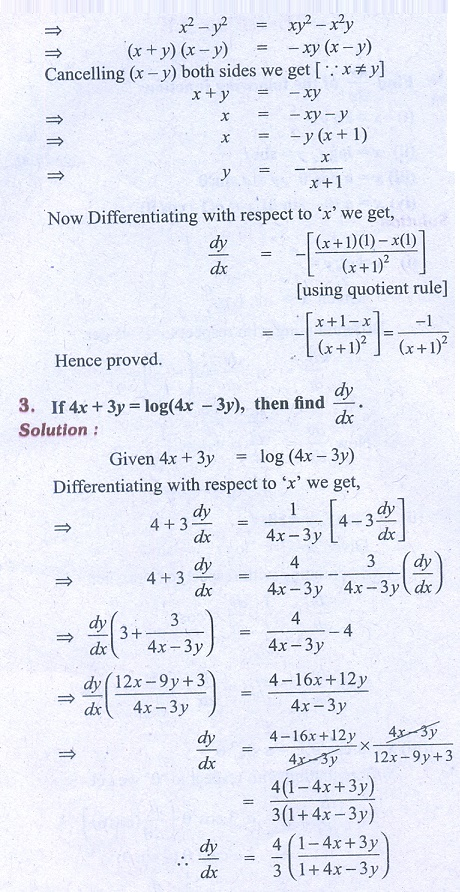Home | | Business Maths 11th std | Exercise 5.6: Derivative of implicit functions

# Exercise 5.6: Derivative of implicit functions

Business Mathematics and Statistics Book back answers and solution for Exercise questions - Mathematics: Differentiation techniques: Differential Calculus: Derivative of implicit functionsTags : Problem Questions with Answer, Solution | Differential Calculus | Mathematics , 11th Business Mathematics and Statistics(EMS) : Chapter 5 : Differential Calculus
Study Material, Lecturing Notes, Assignment, Reference, Wiki description explanation, brief detail
11th Business Mathematics and Statistics(EMS) : Chapter 5 : Differential Calculus : Exercise 5.6: Derivative of implicit functions | Problem Questions with Answer, Solution | Differential Calculus | Mathematics